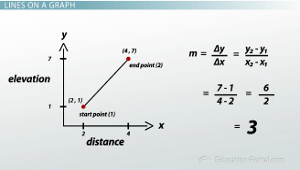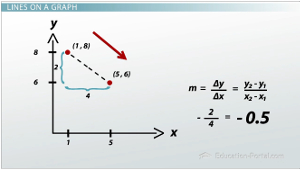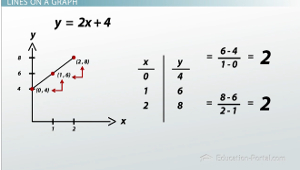# Slopes and Tangents on a Graph

Lesson Transcript
Instructor: Robert Egan
Hit the slopes and learn how the steepness of a line is calculated. Calculate the slopes between points and draw the tangents of curves on graphs in this lesson.

## Hills and SlopeWhich is more of a workout: running in downtown Chicago, or running in downtown San Francisco? Both cities have traffic and some fantastic waterways to enjoy, but San Francisco has very steep hills!

To get an idea of how steep a hill is, it might make sense to look at how much the elevation changes over a set distance. This is known as a slope. So let's say in San Francisco that you're going over a city block that's like Mt. Everest. We can define this with something called a slope. So let's say that we have a hill where the elevation increases 6 feet over a distance of only 2 feet. This sounds like mountain goat territory! In fact, this means that for each foot that we travel forward, we have to go 3 feet up: 6 feet up / 2 feet forward = 3 feet up per 1 foot forward. This is our slope, or, how much the elevation changes over a distance of 1, and I'd say it's very steep.

In Chicago, let's say that the elevation changes 5 feet over a distance of 50 feet. This sounds much more reasonable. Now this means that for every foot you move forward, you only have to go up 0.1 feet, or a little over an inch, because 5 feet up / 50 feet forward = 0.1. Our slope in Chicago is 0.1. We might say that in San Francisco if our slope is 3, it's very steep; it's mountain goat territory. In Chicago, our slope is shallow; it's very small. What happens if we have a negative slope, like -4? Then we would have an elevation change of -4 feet over a distance of 1 foot. That's a steep drop!

An error occurred trying to load this video.

Try refreshing the page, or contact customer support.

Coming up next: Equation of a Line Using Point-Slope Formula

### You're on a roll. Keep up the good work!

Replay
Your next lesson will play in 10 seconds
• 0:06 Hills and Slope
• 2:17 Slope as Lines on a Graph
• 7:16 Tangents
• 9:14 Lesson Summary
Save Save

Want to watch this again later?

Log in or sign up to add this lesson to a Custom Course.

Timeline
Autoplay
Autoplay
Speed Speed

## Slope as Lines on a GraphMathematically, we can tie all of this back to lines on a graph by simply changing feet into coordinate points. So let's look at our steep mountain goat hill. We'll call x the distance along the Earth and y the elevation. Let's say the bottom of the hill is at the point (2,1) where x=2 and y=1. Now our hill elevation will change 6 feet up to y=7 while we move forward 2 feet to x=4. The top of our hill is (4,7). So we're going from the point (2,1) to the point (4,7). In general, you will calculate the slope between two points on a graph such as these. For generality, though, we will write the coordinates of these points as (x sub 1, y sub 1), for the first point, and (x sub 2, y sub 2) for the second point.

The slope, which we will call m, is then defined formally as delta y / delta x. Now delta is a mathematician's way of saying change. So delta y is the change in elevation, and delta x is the change in distance, or how far forward we're going to go. So we can write our slope out as delta y / delta x, or (y sub 2 - y sub 1) / (x sub 2 - x sub 1). We can use this formula to calculate the slope between (2,1) and (4,7). I'm going to call (2,1) my start point and (4,7) my end point. In this case, 2=x sub 1, 1=y sub 1 , 4=x sub 2 and 7=y sub 2. So we find our slope m= (7 - 1) / (4 - 2) = 6 / 2 = 3, exactly as expected!

Let's find the slope of the line connecting (1,8) and (5,6). So, (1,8) is going to be my start point, and (5,6) is going to be my end point. Again plugging in our points, our slope m = delta y / delta x = (y sub 2 - y sub 1) / (x sub 2 - x sub 1) = (6 - 8) / (5 - 1) = -2 / 4 = -0.5, or -1/2. The slope is negative, so the connecting line goes down and to the the right. This is like when I fall down the hill as opposed to the mountain goat traveling gracefully up the hill.To unlock this lesson you must be a Study.com Member.
Create your account

### Register to view this lesson

Are you a student or a teacher?

### Unlock Your Education

#### See for yourself why 30 million people use Study.com

##### Become a Study.com member and start learning now.
Back
What teachers are saying about Study.com
Create an account to start this course today
Used by over 30 million students worldwide